ELECTRICAL FORMULAS AND SUCH

RESISTOR BIDIRECTIONAL CALCULATOR

 resistance calculator black brown red orange yellow green blue violet gray white black brown red orange yellow green blue violet gray white black brown red orange yellow green blue violet gray white gold silver   gold silver none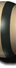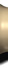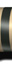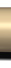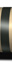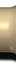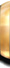K M ±5% ±10% ±20%

Free JavaScripts provided
by The JavaScript Source

4-BAND AND 5-BAND RESISTOR COLOR CHART                                                          OHM'S LAW PIE CHART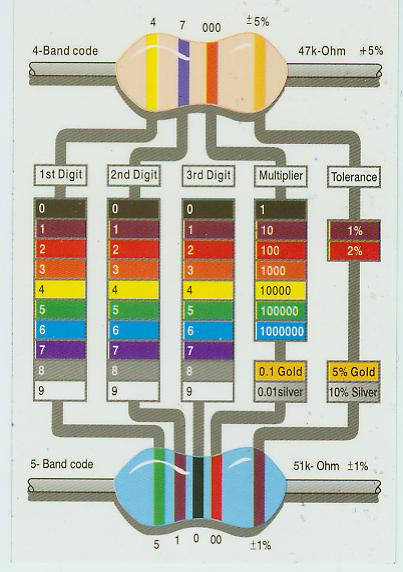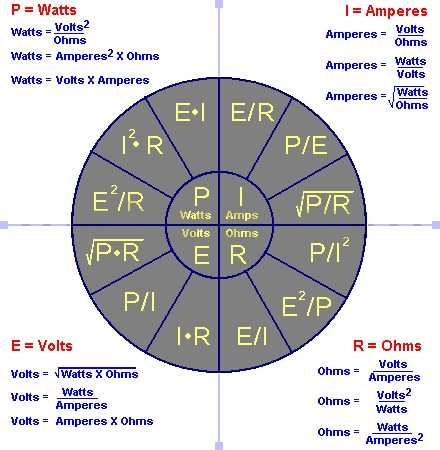0 = BLACK,  1 = BROWN,  2 = RED,  3=ORANGE,  4=YELLOW                                SEE OHMS LAW CALCULATOR BELOW

5 = GREEN,  6 = BLUE,  7 = VIOLET,  8 = GRAY,  9 = WHITE

# Ohms Law Calculator

Enter any two known values and press "Calculate" to solve for the others. For example, a 100 watt light bulb operating on 120 volts AC will have 144 ohms of resistance and will draw 0.833 Amps. Enter 100 in the Watts field and 120 in the Voltage field and press Calculate to find the resistance and current. Fields should be reset to 0 before each new calculation.

Voltage (E) = Current (I) * Resistance (R)
Power (watts) = Current Squared (I^2) * Resistance (R)
Power = I*E = E^2 / R

 Volts (E) Amps (I) Ohms (R) Power (Watts)